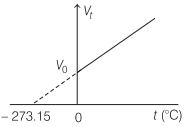# Gas Laws in Physics | Boyle’s Law, Charles’ Law, Gay Lussac’s Law, Avogadro’s Law – Kinetic Theory of Gases

Gas Laws physics:
Through experiments, it was established that gases irrespective of their nature obey the following laws

We are giving a detailed and clear sheet on all Physics Notes that are very useful to understand the Basic Physics Concepts.

## Gas Laws in Physics | Boyle’s Law, Charles’ Law, Gay Lussac’s Law, Avogadro’s Law – Kinetic Theory of Gases

Boyle’s Law is represented by the equation:
At constant temperature, the volume (V) of given mass of a gas is inversely proportional to its pressure (p), i.e.V ∝ $$\frac{1}{p}$$ ⇒ pV = constant

For a given gas, p1V1 = p2V2

Charles’ Law
At constant pressure, the volume (V) of a given mass of gas is directly proportional to its absolute temperature (T), i.e.

V ∝T ⇒ $$\frac{V}{T}$$ = constant

For a given gas, $$\frac{V_{1}}{T_{1}}=\frac{V_{2}}{T_{2}}$$

At constant pressure, the volume (V) of a given mass of a gas increases or decreases $$\frac{1}{273.15}$$ by of its volume at 0°C for each 1°C rise or fall in temperature.
Image
Volume of the gas at t°C,
Vt = V0$$\left(1+\frac{t}{273.15}\right)$$

where, V0 is the volume of gas at 0°C.

Gay Lussac’s or Regnault’s Law
At constant volume, the pressure p of a given mass of gas is directly proportional to its absolute temperature T, i.e.

p ∝ T ⇒ $$\frac{P}{T}$$ = constant

For a given gas, $$\frac{p_{1}}{T_{1}}=\frac{p_{2}}{T_{2}}$$

At constant volume, the pressure p of a given mass of a gas increases or decreases by $$\left(1+\frac{t}{273.15}\right)$$ of its pressure at 0°C for each 1°C rise or fall inVolume of the gas at t°C,
pt = p0$$\left(1+\frac{t}{273.15}\right)$$

where, p0 is the pressure of gas at 0°C.

Avogadro stated that equal volume of all the gases under similar conditions of temperature and pressure contain equal number of molecules. This statement is called Avogadro’s hypothesis. According to Avogadro’s law N1 = N2, where N2 and N2 are number of molecules in two gases respectively.

The number of molecules present in lg mole of a gas is defined as Avogadro’s number.
NA = 6.023 x 1023 per gram mole

(ii) At STP or NTP (T = 273 K and p = 1 atm), 22.4 L of each gas has 6.023 x 1023 molecules.

(iii) One mole of any gas at STP occupies 22.4 L of volume.

Dalton’s Law of Partial Pressure
It states that the total pressure of a mixture of non-interacting ideal gases is the sum of partial pressures exerted by individual gases in the mixture, i.e. p = p1 + p2 + p3 + ………

Kinetic Theory of Gases:
In this concept, it is assumed that the molecules of gas are very minute with respect to their distances from each other. The molecules in gases are in constant, random motion and frequently collide with each other and with the walls of any container.

In this portion, you will learn about the properties of gases, based on density, pressure, temperature and energy. Continue reading here to learn more.